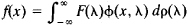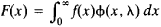# Sturm-Liouville Problem

Also found in: Acronyms.

## Sturm-Liouville problem

[′stərm lyü′vil ‚präb·ləm]
(mathematics)
The general problem of solving a given linear differential equation of order 2 n together with 2 n-boundary conditions. Also known as eigenvalue problem.

## Sturm-Liouville Problem

the problem of finding nonzero solutions of the differential equation

(1) –[p(x)y’]’ + q(x)y = λy

that satisfy boundary conditions of the form

A1y (a) + B1y’ (a) = 0

A2y (b) + B2y’ (b) = 0

and of finding the values of the parameter λ for which these solutions exist. Such solutions are called eigenfunctions, and the corresponding values of λ are called eigenvalues. Under certain conditions on the coefficients p(x) and q(x), the Sturm-Liouville problem can be reduced to the consideration of the analogous problem for an equation of the form

(2) –yq(x)y = λy

The problem was first investigated between 1837 and 1841 by J. Liouville and J. C. F. Sturm.

The solution of some types of equations of mathematical physics by the Fourier method leads to the Sturm-Liouville problem. For example, the problem of the vibrations of a string of uniform density that is fastened at the ends leads to a Sturm-Liouville problem for the equation –y″ = λy with the boundary conditions y(0) = y(π) = 0. In this case there is an infinite sequence of values 12, 22,..., n2,... to which the eigenfunctions sin nx, which form a complete orthogonal system of functions on the interval [0, π], correspond. A similar situation obtains in the general case, which arises, for example, in the study of the flow of heat in a heterogeneous bar. Here too, if the function q(x) in equation (2) is continuous and real on the interval [a, b] and A1B1, A2, and B2 are real numbers, then there exists an increasing sequence of real eigenvalues λ1...,λn,... that tends to infinity. To each λn there corresponds an eigenfunction φn (x) that is defined to an accuracy of a constant factor and that has n zeros on the interval a < x < b. The functions φn(x) form on [a, b] a complete orthogonal system of functions; for equation (1) the eigenfunctions are orthogonal with respect to the weight function p(x).

The completeness of the system of eigenfunctions was proved by V. A. Steklov in 1896. In 1904, D. Hilbert, using the theory of linear integral equations, proved extremely general theorems on the expansion of functions in Fourier series with respect to the system φn(x). As n increases, the eigenvalues and eigenfunctions of the Sturm-Liouville problem for equation (2) tend to the eigenvalues and eigenfunctions for the equation –y″ = λy under the same boundary conditions. The majority of the orthogonal systems of functions encountered in mathematics, such as Legen-dre polynomials and Hermite polynomials, are systems of eigenfunctions of some Sturm-Liouville problems.

Also sometimes called a Sturm-Liouville problem is the boundary value problem of equation (1) under the more general boundary conditions

αiy(a) + βiy’ (a ) + γiy(b ) + δiy’ (b) = 0 i = 1, 2

where αi, βi, γi, and δi, are constants. The most important boundary conditions of this form are y(a) = y(b) and y′(a) = y′(b) (periodic conditions) and y(a) = –y(b) and y′(a) = –y’(b) (semi-periodic conditions).

Many problems of mathematical physics, such as the problem of the flow of heat in an infinite heterogeneous bar, lead to a Sturm-Liouville problem on a half axis or on the entire axis. In the first case, solutions of equation (2) that satisfy the condition A1y(0) + B1y′(0) = 0 are considered; instead of a sequence of eigenfunctions, we have the set of eigenfunctions φ(x, λ), which depend on the continuously changing parameter λ. Instead of an expansion in Fourier series, expansions of the following form are considered:where ρ(λ) is some nondecreasing function. These expansions are analogous to the Fourier integral. HereandA similar situation occurs for the Sturm-Liouville problem on the entire axis.

For some problems of mathematical physics the inverse Sturm-Liouville problem, or the problem of reconstructing the differential equation from the function ρ(λ), is of great importance. This problem, which was posed in a special case by V. A. Ambartsu-mian and in a more general case by the Swedish mathematician G. Borg, was solved by M. G. Krein, I. M. Gel’fand, and B. M. Levitan.

The Sturm-Liouville problem also arises in some problems of quantum mechanics and the calculus of variations.

### REFERENCES

Courant, R., and D. Hilbert. Melody matematicheskoi fiziki, 3rd ed., vol. 1. Moscow-Leningrad, 1951. (Translated from German.)
Sansone, G. Obyknovennye differentsial’nye uravneniia, vol. 1. Moscow, 1953. (Translated from Italian.)
Levitan, B. M. Razlozhenie po sobstvennym funktsiiam differentsial’-nykh uravnenii vtorogo poriadka. Moscow-Leningrad, 1950.
References in periodicals archive ?
The resulting equation is a regular Sturm-Liouville problem. The self-adjoint form of the corresponding linearized dipole equation (22) is
Since a and b are finite, the definition only corresponds to regular Sturm-Liouville problems. We verify that A is a self-adjoint operator with real, countable, and simple eigenvalues [[lambda].sub.n] such that 0 < [[lambda].sub.1] < [[lambda].sub.2] < ...
Klimek, "Fractional Sturm-Liouville problem in terms of Riesz derivatives, Theoretical development and applications of non-integer order systems," in Proceeding of the 7th Conference on Non-Integer Order Calculus and its Applications, Szczecin, Ploand, 2015.
Ghanbari, Similarities of discrete and continuous Sturm-Liouville problems, Electron.
Yang, Existence of nontrivial solutions for a nonlinear Sturm-Liouville problem with integral boundary conditions, Nonlinear Anal.
Note that, discontinuous Sturm-Liouville problems with eigen-dependent boundary conditions and with two supplementary transmission conditions at the point of discontinuity were investigated in [2, 8-11].
Then, by using (2,7) it has been the Sturm identity for Sturm-Liouville problem
The connection between sampling theorems [4, 5] and Lagrange interpolation with nodes from Sturm-Liouville problem spectrum is also discussed in .
of West Bohemia) cover mathematical models, conservation, constitutive laws, classification, types of equations, boundary, initial conditions, liner partial differential equations of the first order, wave equations in one spatial variable, diffusions in one spatial variable, Laplace and Poisson equations in two dimensions, solutions of initial boundary value problems for evolution equations, solutions of boundary value problems for stationary equations, methods of integral transforms, general principles (including the energy method and Laplace equation), Laplace and Poisson equations in higher dimensions, diffusion equations in higher dimensions, and wave equations in higher dimensions, with appendices on the Sturm-Liouville problem and Bessel functions.
Structural properties of the eigenvalues and eigenfunctions of a Sturm-Liouville problem with an impedance boundary condition are studied herein.
Ten of the problems were randomly generated, and another came from a uniform finite-element discretization of the Sturm-Liouville problem

Site: Follow: Share:
Open / Close